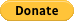# Java : Algorithms and Data StructureThe algorithms and data structures are implemented in Java.

This is a collection of algorithms and data structures I've implemented in my academic and professional life. The code isn't optimized but is written to be correct and readable. The algorithms and data structures are tested and, unless noted, believed to be correct.

## Support me with a donation# What's been implemented:

## Numbers

• Integers
• to binary String
• using divide and modulus
• using right shift and modulus
• using BigDecimal
• using divide and double
• is a power of 2
• using a loop
• using recursion
• using logarithm
• using bits
• to English (e.g. 1 would return "one")
• Longs
• to binary String
• using divide and modulus
• using right shift and modulus
• using BigDecimal
• Complex
• subtraction
• multiplication
• absolute value
• polar value

## String Functions

### String Functions

• Reverse characters in a string
• using additional storage (a String or StringBuilder)
• using in-place swaps
• using in-place XOR
• Reverse words in a string
• using char swaps and additional storage (a StringBuilder)
• using StringTokenizer and additional (a String)
• using split() method and additional storage (a StringBuilder and String[])
• using in-place swaps
• Is Palindrome
• using additional storage (a StringBuilder)
• using in-place symetric element compares
• Subsets of characters in a String
• Edit (Levenshtein) Distance of two Strings (Recursive, Iterative)

### String rotations

• Find in lexicographically minimal string rotation
• Find in lexicographically maximal string rotation

Get A Weekly Email With Trending Projects For These Topics
No Spam. Unsubscribe easily at any time.
java (29,521
algorithm (449
graph (350
data-structures (332
tree (168
sort (84